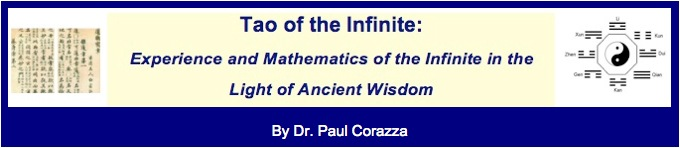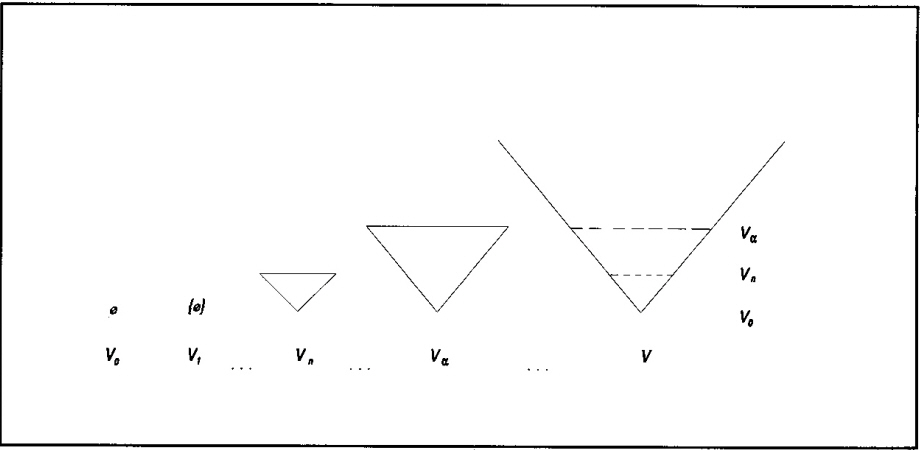Volume 1: The Ultimate Infinite in Mathematics, the Ancient Wisdom, and the Mystical Experience of Totality

Volume 1 introduces the reader to the mathematical infinite and also offers a taste of the Infinite at an experiential level.Two central themes of the volume are:

1. there are many sizes of infinity;
2. the different sizes of mathematical infinity exhibit different properties of the ultimate Infinite: ever bigger infinities are appreciated as better and fuller approximations to the "ultimate Infinite."

Toward the end of the volume, Dr. Wu introduces the most complete expression of the ultimate Infinite known to modern mathematics: the universe of sets, denoted V, which contains within it all possible mathematical objects.To conclude the volume, and set the stage for Volume 2, Dr. Wu takes a closer look at the characteristics and dynamics of the Infinite, as described in the ancient texts, and asks whether the universe of sets in mathematics really exhibits all of them in a natural way. He suggests that perhaps some fundamental dynamics of the Infinite are indeed absent, and these missing characteristics may provide the key to settling a fundamental modern-day research problem in set theory, known as the Problem of Large Cardinals.